# Statistics for Social Science

Lead Author(s): Stephen Hayward

Student Price: Contact us to learn more

Statistics for Social Science takes a fresh approach to the introductory class. With learning check questions, embedded videos and interactive simulations, students engage in active learning as they read. An emphasis on real-world and academic applications help ground the concepts presented. Designed for students taking an introductory statistics course in psychology, sociology or any other social science discipline.

This content has been used by 8,525 students

## What is a Top Hat Textbook?

Top Hat has reimagined the textbook – one that is designed to improve student readership through interactivity, is updated by a community of collaborating professors with the newest information, and accessed online from anywhere, at anytime.

• Top Hat Textbooks are built full of embedded videos, interactive timelines, charts, graphs, and video lessons from the authors themselves
• High-quality and affordable, at a significant fraction in cost vs traditional publisher textbooks

## Key features in this textbookOur Statistics for Social Science textbook allows students to manipulate data, visualize the effects discussed, and explore Lightboard videos that feature instructor explanations to reinforce concepts and calculations.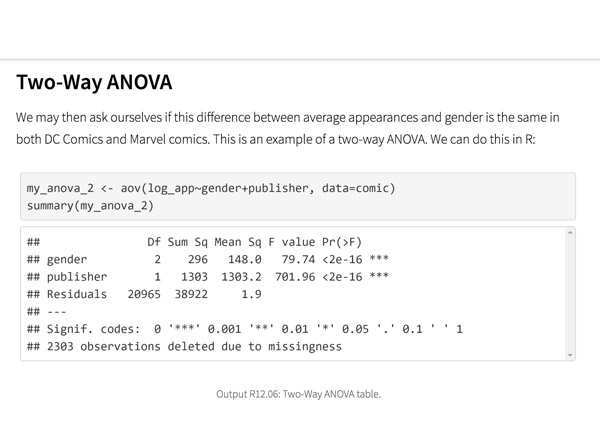Top Hat’s interactive offering includes a complementary module on using R software for data management, graphics, and conducting statistical analyses with examples and practice questions.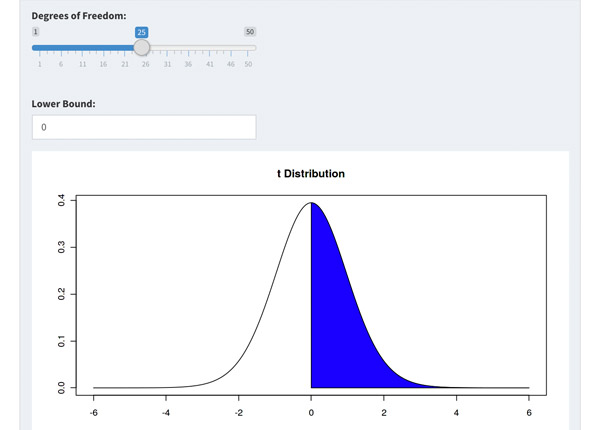Built-in assessment questions embedded throughout chapters so students can read a little, do a little, and test themselves to see what they know!

## Comparison of Social Sciences Textbooks

Consider adding Top Hat’s Statistics for Social Sciences textbook to your upcoming course. We’ve put together a textbook comparison to make it easy for you in your upcoming evaluation.

### Top Hat

Steve Hayward et al., Statistics for Social Sciences, Only one edition needed

### Pearson

Agresti, Statistical Methods for the Social Sciences, 5th Edition

### Cengage

Gravetter et al., Essentials of Statistics for The Behavioral Sciences, 9th Edition

### Sage

Gregory Privitera, Essentials Statistics for the Behavioral Sciences, 2nd Edition

### Pricing

Average price of textbook across most common format

#### Up to40-60%more affordable

Lifetime access on any device

#### $200.83 Hardcover print text only ####$239.95

Hardcover print text only

#### $92 Hardcover print text only ### Always up-to-date content, constantly revised by community of professors Content meets standard for Introduction to Anatomy & Physiology course, and is updated with the latest content ### In-Book Interactivity Includes embedded multi-media files and integrated software to enhance visual presentation of concepts directly in textbook Only available with supplementary resources at additional cost Only available with supplementary resources at additional cost Only available with supplementary resources at additional cost ### Customizable Ability to revise, adjust and adapt content to meet needs of course and instructor ### All-in-one Platform Access to additional questions, test banks, and slides available within one platform ## Pricing Average price of textbook across most common format ### Top Hat Steve Hayward et al., Statistics for Social Sciences, Only one edition needed #### Up to40-60%more affordable Lifetime access on any device ### Pearson Agresti, Statistical Methods for the Social Sciences, 5th Edition ####$200.83

Hardcover print text only

### Pearson

Gravetter et al., Essentials of Statistics for The Behavioral Sciences, 9th Edition

#### $239.95 Hardcover print text only ### Sage McConnell, Brue, Flynn, Principles of Microeconomics, 7th Edition ####$92

Hardcover print text only

## Always up-to-date content, constantly revised by community of professors

Constantly revised and updated by a community of professors with the latest content

### Top Hat

Steve Hayward et al., Statistics for Social Sciences, Only one edition needed

### Pearson

Agresti, Statistical Methods for the Social Sciences, 5th Edition

### Pearson

Gravetter et al., Essentials of Statistics for The Behavioral Sciences, 9th Edition

### Sage

Gregory Privitera, Essentials Statistics for the Behavioral Sciences, 2nd Edition

## In-book Interactivity

Includes embedded multi-media files and integrated software to enhance visual presentation of concepts directly in textbook

### Top Hat

Steve Hayward et al., Statistics for Social Sciences, Only one edition needed

### Pearson

Agresti, Statistical Methods for the Social Sciences, 5th Edition

### Pearson

Gravetter et al., Essentials of Statistics for The Behavioral Sciences, 9th Edition

### Sage

Gregory Privitera, Essentials Statistics for the Behavioral Sciences, 2nd Edition

## Customizable

Ability to revise, adjust and adapt content to meet needs of course and instructor

### Top Hat

Steve Hayward et al., Statistics for Social Sciences, Only one edition needed

### Pearson

Agresti, Statistical Methods for the Social Sciences, 5th Edition

### Pearson

Gravetter et al., Essentials of Statistics for The Behavioral Sciences, 9th Edition

### Sage

Gregory Privitera, Essentials Statistics for the Behavioral Sciences, 2nd Edition

## All-in-one Platform

Access to additional questions, test banks, and slides available within one platform

### Top Hat

Steve Hayward et al., Statistics for Social Sciences, Only one edition needed

### Pearson

Agresti, Statistical Methods for the Social Sciences, 5th Edition

### Pearson

Gravetter et al., Essentials of Statistics for The Behavioral Sciences, 9th Edition

### Sage

Gregory Privitera, Essentials Statistics for the Behavioral Sciences, 2nd Edition

## About this textbook

### Lead Authors

#### Steve HaywardRio Salado College

A lifelong learner, Steve focused on statistics and research methodology during his graduate training at the University of New Mexico. He later founded and served as CEO of Center for Performance Technology, providing instructional design and training development support to larger client organizations throughout the United States. Steve is presently lead faculty member for statistics at Rio Salado College in Tempe, Arizona.

#### Joseph F. Crivello, PhDUniversity of Connecticut

Joseph Crivello has taught Anatomy & Physiology for over 34 years, and is currently a Teaching Fellow and Premedical Advisor of the HMMI/Hemsley Summer Teaching Institute.

## Explore this textbook

Read the fully unlocked textbook below, and if you’re interested in learning more, get in touch to see how you can use this textbook in your course today.

# Normal Probability DistributionsThe beams holding up the ceiling and roof of this church in California are shaped like normal distributions.​​ ​​

## Chapter Objectives

After completing this chapter, you will be able to:

• Describe the relationship between scores on a normal distribution
• Determine the probability associated with a z-score using the Standard Normal Table
• Determine the z-score associated with a probability in the Standard Normal Table
• Convert a z-score to a raw score
• Create a distribution of sample means by using the central limit theorem
• Determine the probability of a given sample mean
• Substitute the normal distribution for the binomial distribution

## Introduction

Normal probability distributions allow us to make inferences about all kinds of data. Many variables of interest to social scientists, such as scores on standardized tests (e.g., SAT, GRE), many biological measurements (e.g., weight), and even financial indices (e.g., interest rates) are normally distributed. Mathematicians have determined that normal distributions follow regular patterns, and by knowing these characteristics, we can exploit them to make inferences about data. As we will see, any raw measure can be converted to a “z-score” so that a single table, called the Standard Normal Table, can be used to find the proportion of a population below a certain raw measure. We can also use the Standard Normal Table to determine the z-score that marks off a certain proportion of a population, such as the uppermost 10%. This knowledge can also be applied to distributions of possible sample means, so that the probabilities associated with individual sample means can be found, laying the basis for testing hypotheses about sample means that will be covered in later chapters. Finally, the normal distribution can also be used in place of the binomial distribution to determine probabilities when there are large numbers of observations. This shortcut greatly simplifies the calculations needed in these situations without sacrificing much accuracy.

## The Normal Curve as a Chart of the Distribution

Many variables in which social scientists are interested have a frequency distribution shape that is familiar…a bell curve. Intelligence tests, errors made in measurement, and the weights of machine-filled commercial products (think bags of potato chips) are just some examples. These raw measures (e.g. weight in lbs or kgs, number of chips in a bag, score on an intelligence test, etc.) can be thought of as a raw value. That value’s position on the chart indicates where that particular data point is relative to the others, both in terms of the value itself, but also in terms of frequency, such as how many people in our sample or population have that same value.

Example: The ACT Test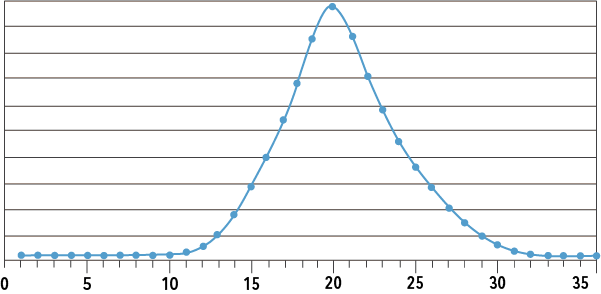Figure 6.1: ACT scores​

The ACT is a test intended to measure academic achievement in high school and is used by colleges (mainly in the central U.S.) in the admissions process.  Figure 6.1 presents the scores on the ACT in 2005 for U.S. high school students.

Scores on the ACT in 2005 showed a bell-shaped distribution. In this type of distribution, more people have scores that are close to the mean, and fewer people have scores far from the mean. We can use the normal curve to understand the relationship of any single person’s score to others in the same distribution. Normal curves portray situations where there are many observations around some central point or measurement, with decreased observations the further the value is from the central point.

Example: Arrival to ClassFigure 6.2: Relative arrival times to class in minutes. 0 is class starting time​​

A chart of the times students enter a classroom may also show this pattern. The chart shows a possible distribution of arrival times relative to the start of a class. Few students arrive more than 6 minutes early, and the number of arrivals increases as the class time approaches, with most arriving just two minutes before the start of class. From that peak, the number of students entering the class decreases, with very few students arriving more than three minutes late. The chart tells us that although arrival times that are very early or very late are rare (not many people arrive at these times), most students arrive before those who are very late.

6.01

Where in a normal curve can most observations be found?

A

The left tail

B

The center

C

The right tail

6.02

A normal curve portraying the weights of machine-filled potato chip bags is centered around 16 ounces, which is the manufacturer’s target weight. Any other weight is an error in production. Knowing what we know about a bell curve, which of the weights below will occur more frequently than the others?

A

15

B

13

C

11

## The Normal Curve as a Chart of a Variable

As you see, much information can be inferred from such a chart. This analogy of the normal curve as a chart or map can help to understand any variable that is normally distributed. Learning how to read this kind of chart allows us to make inferences about the variable. The distribution chart for any variable will have the values and units of that variable on the x-axis and the frequency of each score on the y-axis. For any particular value of a variable, the normal distribution tells us how common the value is (its frequency) and how far it is from the mean (above or below, as well as how far). It also shows the proportion of the distribution that is above or below that value. How far a value is from the mean can be expressed in the units of the variable (the x-axis labels) or in standard deviations. It is also important to remember that the distribution contains all of the values that make up the population.

Video Example: Temperature Anomalies

The video shows how temperature anomalies (the difference from the average temperature to the actual temperature) are normally distributed, and how the distribution has changed over time.

Example: IQ Scores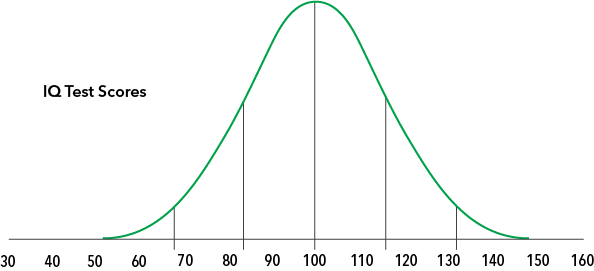Figure 6.3: IQ test scores​​

This figure shows the population of scores on a certain IQ test which, in this example, has a mean of 100 and a standard deviation of 16. The vertical lines are each one standard deviation apart. It can be seen that an IQ score of 100 is in the center and has the most people near it. The scores can be said to “cluster” about the mean. As IQ scores differ from this central landmark, either above or below, there are increasingly fewer people with those IQ scores. There are fewer people with a score of 90 than there are with a score of 95, and even fewer people with a score of 70. Try answering the questions below using the IQ score chart.

6.03

Click the score that is two standard deviations above the mean.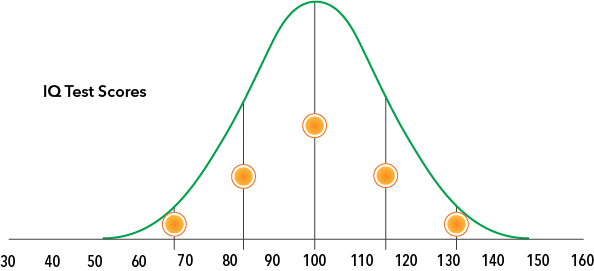6.04

Click the score that is one standard deviation below the mean.6.05

Which of these IQ scores occurs the least?

A

100

B

90

C

120

D

140

## Characteristics of the Normal Curve

There actually is no single normal curve. Variables have different means and standard deviations, which define the distribution and give it its particular shape. The figure below shows four normal distributions, each with a unique combination of mean μ (“mu”) and standard deviation σ (“sigma”). The mean defines where the center of each distribution will be, and the standard deviation defines the spread (or width) of the distribution. Narrower distributions, with the values tightly clustered around the mean, have smaller standard deviations. Wider distributions have larger standard deviations because they have more variability among the values, making them appear more spread out. Remember that a standard deviation is the square root of the variance (σ=√σ2).Figure 6.4: Normal curves with differing variances​

Therefore, as a frequency distribution is defined by its mean μ and standard deviation σ, there can be many different examples of curves that can be described as “normal,” and they all share certain characteristics. Normal curves get their shape because the characteristics being measured tend to be more similar across people than different.

### Normal Distributions in Everyday Life

The video shows how normal distributions are evident in unexpected places.

### Three Properties of Normal Curves

The common shape of normal curves is derived from the following properties:

• Normal curves are symmetric about the mean.
• All three measures of central tendency are the same in a perfect normal curve.
• The proportion of the areas between the standard deviations are known.

## Symmetry and Central Tendency

These three properties create a family resemblance among the distributions of all variables that are normally distributed. In all normal curves, the right and left halves are mirror images of each other. Each half has the same shape and slope but is reversed. In perfect normal curves, knowing that the mode, median, and mean are all the same value is useful in that if one measure is known, then the other two measures are also known. Since we don’t live in a perfect world, however, we can take this to mean that the three measures of central tendency can be expected to be approximately the same in a normal distribution. Furthermore, as all three measures are the same value, that single value also has the properties of all three measures of central tendency. The mean of a normal distribution is also the center score (the median), with half the observations greater than it, and half less than it. Being the middle score is not regularly a property of the mean, but as it is the same value as the median, that property is assumed by the mean, so it can be stated in that way.

### Areas Under the Normal Curve

Finally, in any normal curve, the sections of the total area defined by the standard deviation are the same regardless of the value of the standard deviation. That is, the sizes of the areas marked off by the standard deviations are the same for every normal distribution. The exact proportions of these areas can be determined with geometry (we will not detail this part, but thank the mathematicians for their work), and are shown in the figure below.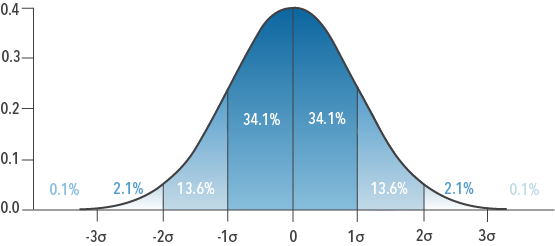Figure 6.5: Areas under the normal curve​

As the figure shows, 34.1% of the distribution is between one standard deviation above the mean and the mean itself. This is also the percentage of the distribution that is between the mean and one standard deviation below it. There are 13.6% of the scores between one standard deviation above the mean (1σ in the figure) and two standard deviations above the mean (2σ in the figure). These percentages are the same for all normal distributions. Recall that a normal distribution represents all scores. As normal curves all share the same proportions, we know that 50% of scores in all normal distributions will be either above or below the mean. The sizes of the areas sectioned by the standard deviation in any normal curve will sum to 100%, as it does above.

### Examples: Normal Curve Areas

Example 1: Exam Grades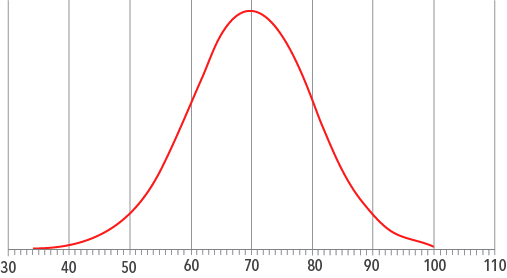Figure 6.6: Distribution of exam scores​​

As part of an ongoing study, imagine that the psychology department has kept detailed data on student performance. The figure presents the population of grades on Exam 1 of a PSY 100 course over ten years. This set of exam grades is normally distributed, with a mean μ of 70 and a standard deviation σ of 10.

Although the mean and standard deviation for the set of exam grades is not the same as for the IQ test (which were 100 and 16, respectively), the shape of the distribution is similar. This is because both are normally distributed, and while they may vary some in appearance, they share all of the properties of a normal curve. First, the distribution of exam grades is symmetric about the mean of 70: each half is a mirror image of the other. Second, all three measures of central tendency will be the same value, or at least very close to each other. The mean of 70 is also the mode, as it can be seen that 70 is the most frequently occurring grade. And, as the mean is also the median in a normal distribution, 50% of the grades fall above (or below) 70. We also know that 34.1% of the grades will be between one standard deviation and the mean, so 34.1% of the exam grades are between 60 (one standard deviation below the mean) and 70. The same percentage is also between 80 (one standard deviation above the mean) and 70. Another way to think about this is that 95.4% of the grades fall between 50 and 90 (or ±2σ from the mean).

Example 2: Tree HeightsFigure 6.7: Distribution of tree heights​​

Tree heights tend to be normally distributed. The distribution of heights of a certain species of tree shows the heights are normally distributed with mean μ of 25 meters and a standard deviation σ of 2 meters. The distribution is symmetric about the mean of 25, and all three measures of central tendency are the same value, 25. We also know that 34.1% of the heights will be between one standard deviation and the mean, so 34.1% of the heights are between 23 (one standard deviation below the mean) and 25. The same percentage is also between 27 (one standard deviation above the mean) and 25.

6.06

What area is the mirror image of the area marked scores of 115 and 130?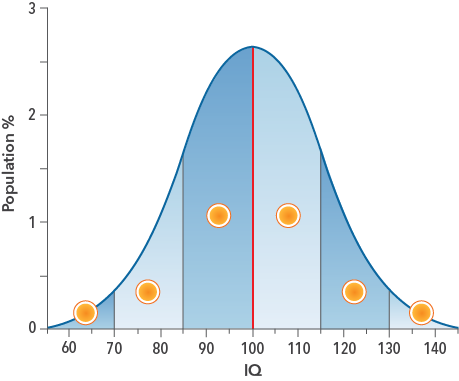6.07

What areas make up the lowest 15.8% of the distribution?6.08

In a normally distributed population, what percentage of scores fall below the mode?

## Normal Curve and z-Score Review

You probably have noticed the consistency in the general shapes of the normal curves and patterns of the standard deviations in the figures so far. The means and standard deviations are constant landmarks in a normal distribution despite the different values on the x-axes. This makes the z-score a valuable tool in locating a raw score in a distribution. Recall that a z-score converts any individual raw value to a score that tells us where and how far that score is from the mean in terms of the standard deviation.

### Standard Normal Distribution

Z-scores follow a special normal distribution with a mean of 0 and a standard deviation of 1 called the standard normal distribution. The standard normal distribution makes it easier to apply the properties of the normal distribution to data collected on different scales. Rather than create and label a normal curve for every combination of mean and standard deviation, we can simply convert the raw scores to z-scores and use the standard normal distribution. Combining z-scores with properties of the normal distribution will allow us to know not only where a score is relative to the mean of the distribution, but also how much of the distribution falls below or above it.

### Examples

Example 1: U.S. Male Height Data

Schilling, Watkins & Watkins (2002) used data from surveys conducted by the US National Center for Health Statistics from 1988-1994 to determine that the mean height in inches for males aged 20-29 was 69.30, with a standard deviation of 2.92. The distribution of heights was also normally distributed. Using this data, we can determine the z-score for a male whose height is 75.14 inches:

The z-score for this person is +2.00, telling us that his height is two standard deviations above the mean. Using what we know about areas marked by the standard deviation in the standard normal distribution, it can be seen that this person’s height is greater than a majority of other males’ heights as much of the normal curve is to the left of the z-score of +2.00. By adding the areas of the curve from the chart for each of the sections below this z-score, we can see that 97.8% of males will have a height less than this person. Summing the percentages of the sections above the z-score tells us that 2.2% of the distribution is taller than this person. Thus, by using the standard normal distribution, we can learn not only where a score is relative to the mean, but the proportions of the distribution relative to this person.Figure 6.8: Areas under the normal curve​

Example 2: U.S. Male Height Data

Consider the height of a male from the same population whose height is 66.38 inches. The population was normally distributed, with μ = 69.30 inches and σ = 2.92 inches. The z-score for this individual can be found using the z-score formula:

The z-score for this person is -1.00, putting his height one standard deviation below the mean. Summing the percentages for the sections below this z-score tells us that he is taller than 15.8% of the distribution. We can also see that 34.1% of the distribution is between his z-score and the mean.

6.09

What is the z-score for a male whose height is 63.46 inches?

6.10

What is the z-score for a male whose height is 78.06 inches?

## Introducing the Standard Normal Table

The standard normal distribution can be used to determine what proportion of a distribution lies above or below a particular individual’s z-score. However, the figure is useful only when we have whole number z-scores. The information we have learned so far cannot be used for z-scores that have decimals. Consider a male whose height is 70.51 inches. Using the population information for males from Schilling, et al. (2002):

As the z-score for this height is not a whole number, the figure lacks the precision we need to locate this score on the distribution. Fortunately, mathematicians and statisticians have solved this problem for use with the Standard Normal Table (below), which presents the areas under the normal curve with sufficient precision for our needs. The Standard Normal Table lists the areas associated with z-scores between -3.49 and +3.49. While the distribution is theoretically not closed at either end, the probability of an individual’s score being more than 3.49 standard deviations from the mean (that is, z-scores less than -3.49 or greater than +3.49) is so small, that in most cases we can treat it as 0.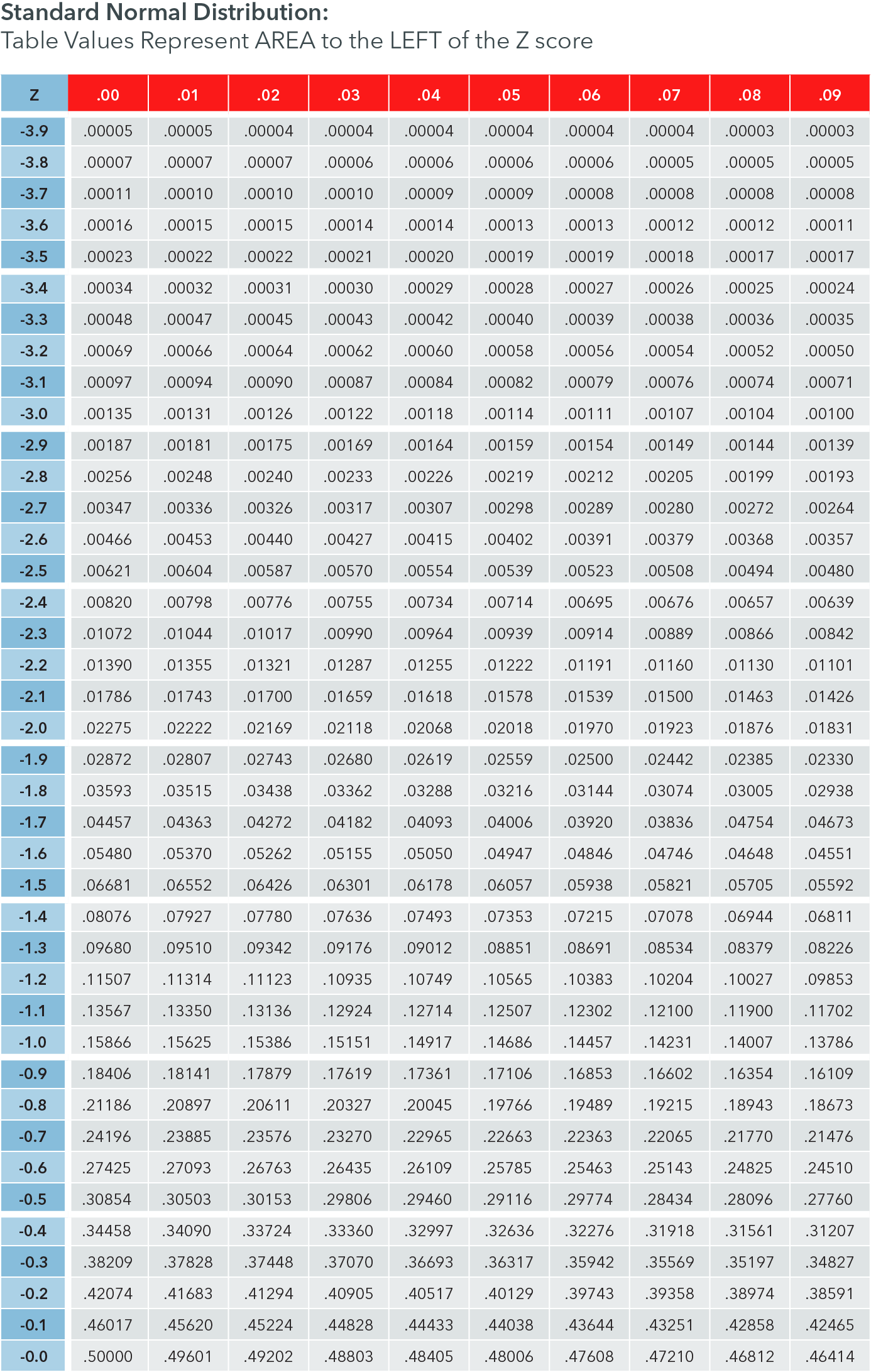Figure 6.9: Standard normal probabilities associated with negative z-scores​​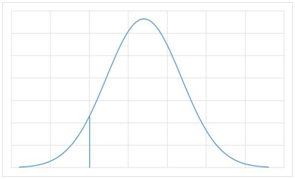Figure 6.10: Normal distribution sectioned by a z-score​

Each z-score divides the distribution into two distinct sections. In this figure, a normal distribution is divided into two parts by a negative z-score. The size of each section depends on the value of the z-score. As the z-score gets smaller, the section to the left will get smaller; as the z-score gets larger, the section to the left will get larger. The size of the section to the right of the z-score also changes in relation to the value of the z-score. As the section to the left gets smaller, the section to the right must get bigger, and vice versa. The Standard Normal Table gives the size of the section that is to the left of, or below, each z-score. The rows and columns in the table are used for each z-score, and the entries are the proportions of the normal distribution that are to the left of the z-score. The proportions are expressed to four decimal places, which again increases the precision of our knowledge. The proportions can be converted to percentages of the total distribution by moving the decimal two places to the right. A proportion of .1685 can also be expressed as 16.85%.

### Finding the Area Below a z-ScoreFigure 6.11: Locating the probability associated with z = -0.91 in the Standard Normal Table​​​​

A portion of a Standard Normal Table is presented above. Suppose that someone on the PSY 100 exam received a grade of 79. We know the grades are normally distributed with a class average of 84 and a standard deviation of 5.5, we can calculate a z-score of -0.91. The Standard Normal Table enables us to find the proportion of people who scored less than this person. To use the table for a z-score of -0.91:

• Locate the z-score in tenths in the left column. This is the row labeled -0.9 for our score.
• Move across to the right to the column with the correct hundredths value. For our score, this is the 0.01 column
• The value at the intersection of the row and column is the area to the left of a z-score of -0.91 in the normal distribution curve.

Therefore, a z-score of -0.91 cuts off an area to the left of the z-score of .1814. This means that 18.14% of the exam grades are less than this person’s grade. More importantly, this also tells us the probability of a grade lying in the area to the left of a z-score of -0.91 is .1814.

Since the area under the curve also equals the probability of obtaining a score in that range by random selection, we can infer that the probability of obtaining such an extreme score or less is approximately .1814 or ~ 18%.

### Finding the Area Above a z-Score

Notice that this also tells us how many people scored above a z-score of -0.91. Since the normal distribution represents all possible scores, (100% or a proportion of 1.0000), we can subtract the area to the left of any z-score found in the Standard Normal Table from 1.0000 to obtain the area above that z-score. For the z-score of -0.91:

This means that 81.86% of all the grades are greater than the grade that has a z-score of -0.91.

### Finding the Area Between a z-Score and the Mean

We also use what we have determined from the Standard Normal Table to learn how much of the normal distribution is between the z-score and the mean. As the mean is also the median, 50% of the distribution lies below or above the mean. Thus, using the same logic as we did above, we can subtract the area given in the Standard Normal Table for the z-score of -0.91 from .5000 to obtain the area between the z-score of -0.91 and the mean.

This means that 31.86% of the exam grades are between the population mean and the grade that has a z-score of -0.91.

### Examples

Example 1: Cell Phone Batteries

Assume that a test of a company’s cell phone batteries has found that the mean number of charge cycles for the brand is 400, with a standard deviation of 150. The company wishes to determine how many of their phones will last for at least 600 charge cycles. To determine the percentage of batteries that will last for at least 600 charge cycles, we first need to convert 600 charge cycles to a z-score by using the z-score formula.

The z-score is +1.33, which tells us that 600 charge cycles is exactly 1.33 standard deviations above the mean of 400. We can now use the Standard Normal Table to see how this z-score divides the distribution. The relevant section of Standard Normal Table is presented here.

• Locate the z-score in tenths in the left column. This is the row labeled 1.3 for our score of +1.33.
• Move across to the right to the column with the correct hundredths value. For our score, this is the 0.03 column
• The value at the intersection of the row and column is the area to the left of that z-score in the normal distribution curve.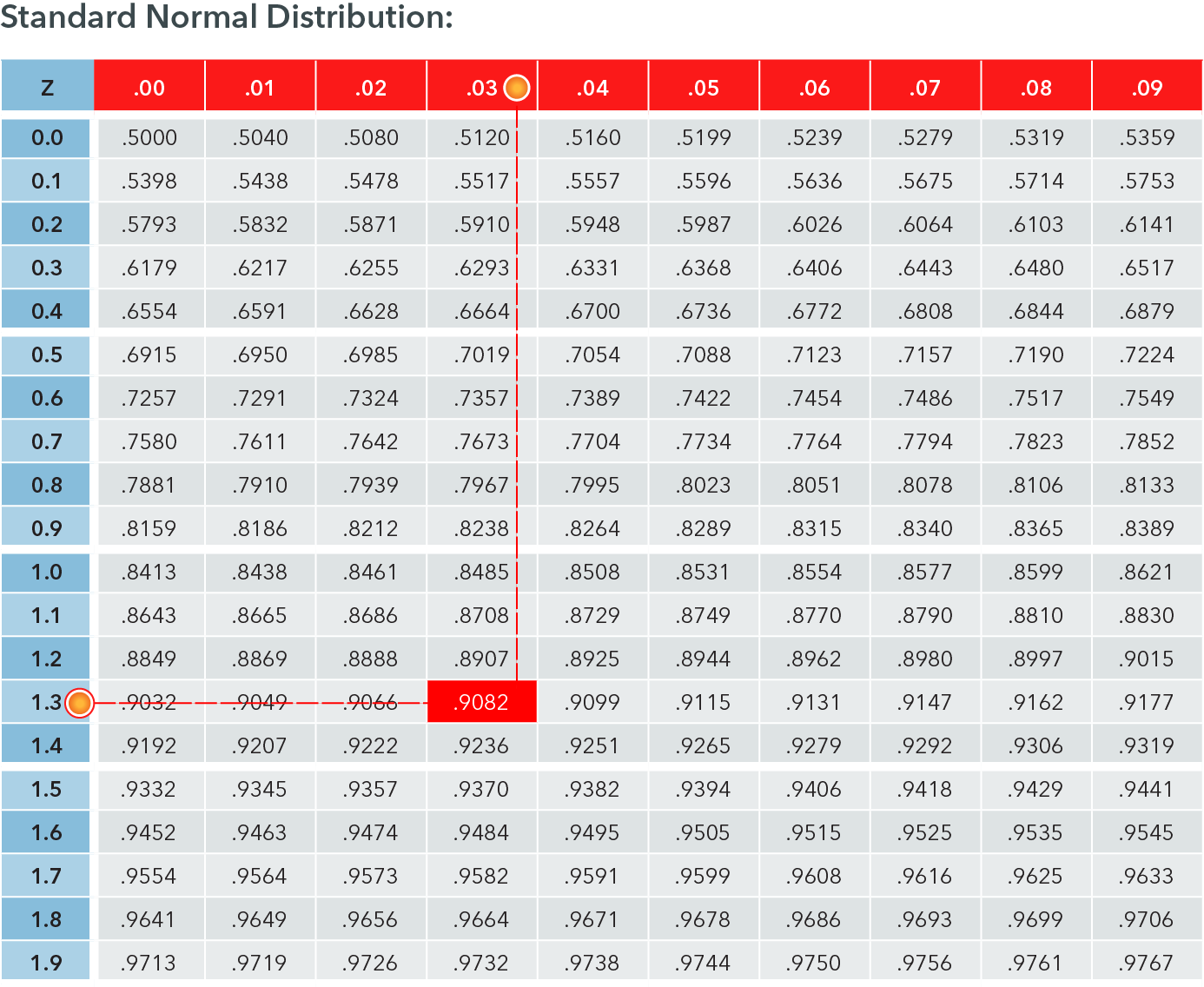Figure 6.12: Locating the probability associated with z = +1.33 in the Standard Normal Table​​

The proportion of the distribution that is below the z-score of +1.33 is .9082. We can say that 90.82% of the batteries will charge for 600 cycles or fewer.

To find the proportion that will charge for at least 600 cycles, we need the area above the z-score. Recall that we can subtract the area to the left of the z-score from 1.0000 to obtain the area above that z-score. For this example, for a cycling charge of 600 and corresponding z-score of +1.33:

Since the area under the curve also equals the probability of obtaining a score in that range, we can infer that the probability of randomly selecting a cell phone battery that will charge for at least 600 cycles is approximately 9.18%.

Example 2: Cell Phone Batteries

We also can learn about how much of the population is between the z-score and the mean. As we learned earlier, 50% of the distribution lies below or above the mean. Thus, using the same logic, we can subtract the area to the left of the mean (50% or 0.5000) from the value given in the Standard Normal Table for a z-score to obtain the area between that z-score and the mean. For this example of a z-score of +1.33:

The company can use this determine that 40.82% of the cell phone batteries that they sell will charge for between 400 and 600 charge cycles.

6.11

Click the table entry that shows the proportion of the normal curve that is below z = +0.96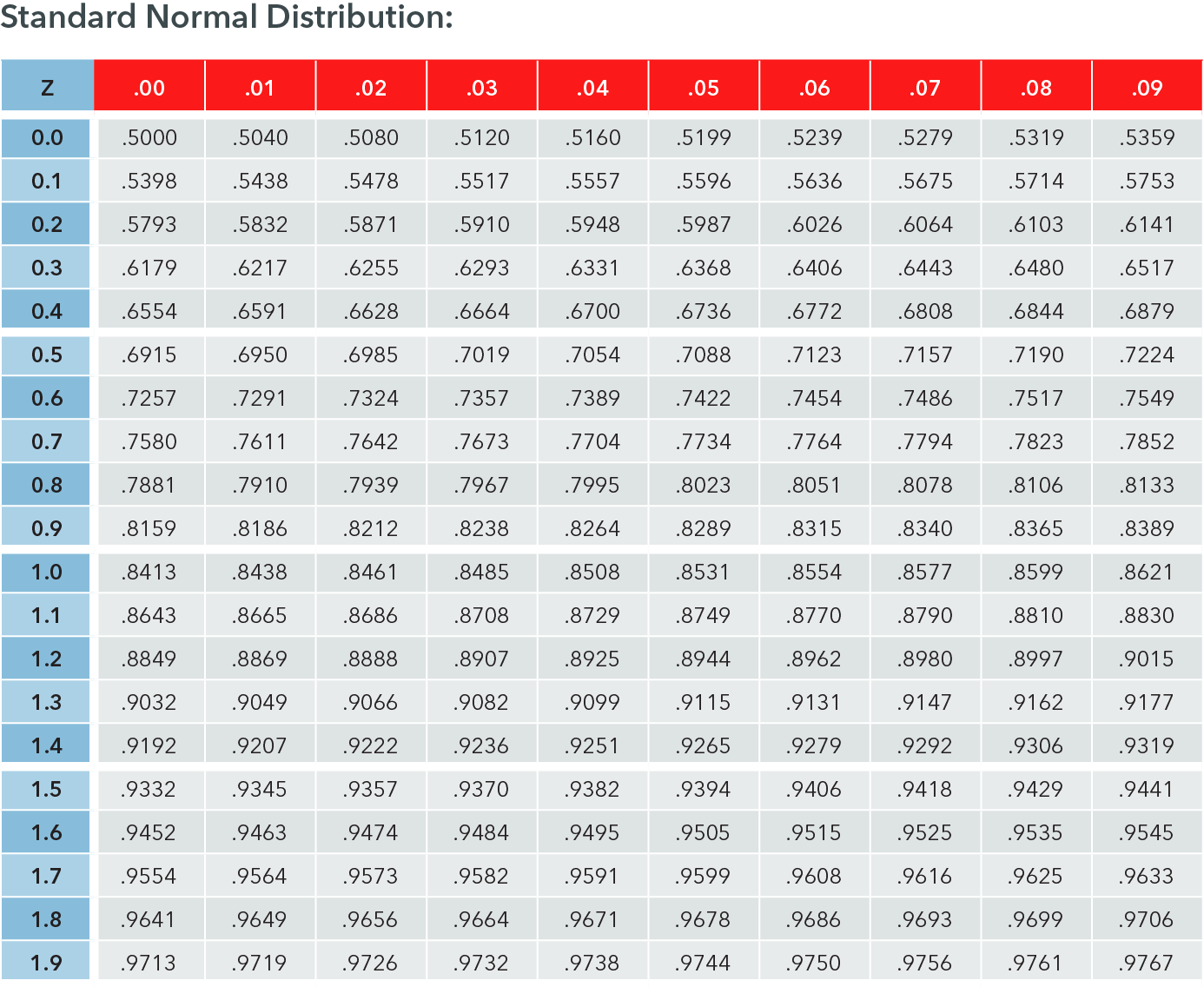6.12

What proportion of scores falls below x = 25 in a normal distribution where $\mu$ = 65 and $\sigma$ = 12? Round your answer to four decimal places.

6.13

Which areas of a normal distribution can be found using subtraction after a value from the Standard Normal Table has been found?

A

The area to the left of a $z$-score

B

The area to the right of a $z$-score

C

The area between the mean and a $z$-score

## Using the Standard Normal Table to Find z-Scores.

The Standard Normal Table can also be used in reverse. A table entry can be selected, and then the row and column headings can be combined to determine the z-score. This can be useful if there is a particular proportion or percentile needed. The table entries can be scanned for the entry closest to the one that is needed. As the mean is also the median, with half the scores above or below it, the z-score marking off any proportion lower than 0.5000 will have a z-score that is negative. Similarly, any proportion above 0.5000 will have a positive z-score. Note that the proportions in the Standard Normal Table above vary with the z-score, with the smallest entries at the top of each table. Remember that each z-score divides the distribution into two sections, but the table entries in the Standard Normal Table presented above are for the left section only.

### Examples

Example 1: U.S. Female Heights Data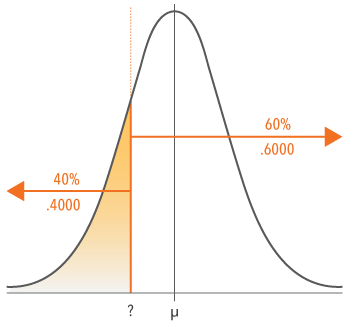Figure 6.13: Heights of women aged 20-29 years​

Schilling, Watkins, & Watkins (2002) also used data from the U.S. National Center for Health Statistics surveys to examine the heights of women aged 20-29. They determined that the mean height for women was 64.10 inches with a standard deviation of 2.75. What heights of women are in the lowest 40% of the population? This area is marked off on the normal distribution chart. The mean female height, 64.10 serves as our landmark in the middle. The orange line marks off the lower 40% of the population. It is to the left of the mean as we are looking for the lowest 40 percent–remember that the mean is the 50% landmark. The relevant section of Standard Normal Table is presented here.

• Scan the table to find the proportion that is closest to .4000 (40%). The entry that is closest is .4013.
• Look at the row heading for this value to obtain the z-score to the tenths place. Here, it is -0.2.
• Look at the column heading for this value to obtain the z-score to the hundredths place. Here, it is 0.05.
• Combine these values for the z-score of -0.25.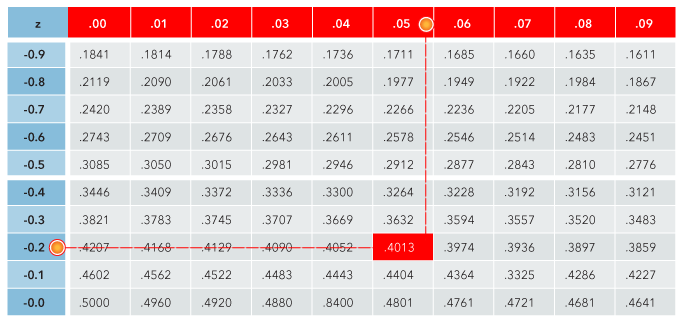Figure 6.14: Locating the probability associated with z = -0.25 in the Standard Normal Table​​​

This tells us that a z-score of -0.25 is the one that divides the distribution into the sections that are needed here. The area to the left of the z-score of -0.25 is 40.13% of the entire distribution. As it is close, we could round this to 40%. Therefore, any female height with a z-score -0.25 will be in the lower 40% of the distribution. Since we think of height in inches and not z-scores, we can convert the z-score of -0.25 back to the units of height by using the equation for calculating the z-score in reverse. We will present this in the next section.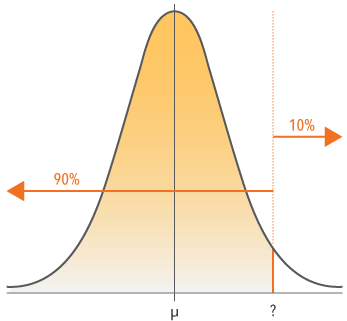Figure 6.15: GPAs of Graduating Students​

Example 2: Honors Recognition

The faculty in a sociology department wish to provide honors awards to graduating students whose grade point averages (GPA) are in the top 10%. To find this, they need to find the z-score that divides the distribution such that 10% is above the z-score. This area is marked off on the normal distribution chart. As the distribution represents 100% of the GPAs, the same z-score that has 10% above it will have 90% below it. The z-score is to the right of the mean because the mean marks off the top 50% of the distribution. Therefore, the top 10% will also be to the right of the mean, and thus we are looking for a positive z-score. The relevant section of the Standard Normal Table is presented here. Remember that the Standard Normal Table lists the proportions to the left of, or below each z-score, so we must find the z-score that divides the chart so that the section to the left is 90%: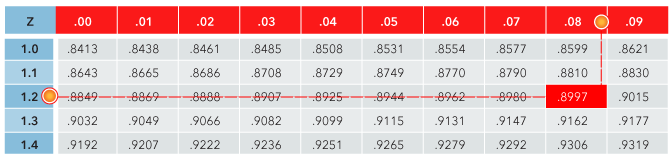Figure 6.16: Locating the Probability Associated with z = +1.28 in the Standard Normal Table​​
• Scan the table to find the proportion that is closest to .9000 (90%). The entry that is closest is .8997.
• Look at the row heading for this value to obtain the z-score to the tenths place. Here, it is +1.2.
• Look at the column heading for this value to obtain the z-score to the hundredths place. Here, it is 0.08.
• Combine these values for the z-score of +1.28.

The area to the left of the z-score of +1.28 is .8897 or 89.97% of the entire distribution. As it is close, we could round this to 90%. The z-score +1.28 divides GPAs such that 90% of the distribution is below this point and 10% are above this point. Any student whose GPA has a z-score greater than +1.28 will qualify for honors recognition.

6.14

Click on the row and column headings for the table entry of .9699.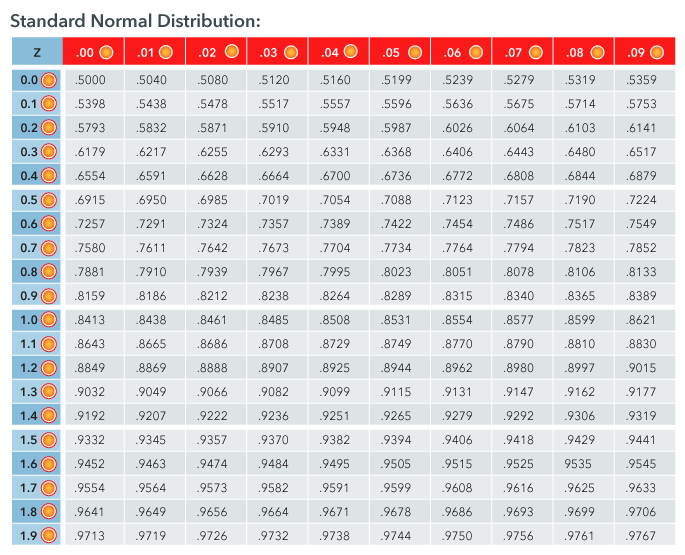6.15

Click on the row and column headings for the table entry of .9474.6.16

Click on the row and column headings for the table entry that is closest to .8500.## Z-Score to Raw Score Conversion

The Standard Normal Table can be used to find the z-score that divides a normal distribution into two sections of known areas. However, it is often more useful to know the raw score that corresponds to that z-score. To convert a z-score to a raw score requires that we use the formula for the z-score presented earlier. It is:

When we have used this formula earlier, we knew three of the four parts. The raw score (x), the mean (μ) and the standard deviation (σ) were known, and the equation was solved for a z-score. We can also use this same formula to find the raw score x that corresponds to a z-score, provided we also know the mean μ and standard deviation σ. Again, if we know three pieces of the formula, we can solve for the fourth. To simplify the math, however, it is preferable to use a transformation of the z-score formula.

### Examples

Example 1: U.S. Female Heights Data

An earlier example delineated the bottom 40% of the distribution of heights of U.S. females. Since we don’t usually think of height in units of z-score, it is useful to convert the z-score back into units of height (inches). We know that heights are normally distributed, with a population mean of 64.1, and a standard deviation of 2.75. Filling in the formula with what we know, we get:

Based on this data, we know that a woman who is 63.4 inches tall is in the bottom 40th percentile of the U.S. population. That is, we know that she is taller than 40% of all U.S. women.

Example 2: Honors Recognition GPA

A previous example found that the z-score +1.28 delineated the top 10% of the distribution of GPAs for graduating sociology students. To make it easier for the faculty to identify which students are in this group, the z-score should be converted to a GPA. The graduating students from the department have GPAs that are normally distributed, with a population mean of 3.05, and a standard deviation of 0.40. Filling in the formula with what we know, we get:

The faculty can use a GPA of 3.562 to determine which students are in the top 10% of the graduating class. Notice that this also tells us that 90% of all the GPAs for graduating seniors are below this point.

Example 3: Vocabulary Skills

A middle school teacher wants to offer vocabulary skills training to students who score poorly on a reading ability assessment. Research has demonstrated that the intervention is most appropriate for children who score in the lowest 35% of the assessment. A z-score of -0.38 marks off the lower 35% of a normal distribution. What reading ability score will be the cutoff for the assessment? Assume the reading ability scores are normally distributed with μ = 65 and σ = 7.

Students with a reading ability assessment less than 62.34 are in the lower 35% of the distribution and should be offered the intervention.

6.17

Which of the following do we not need to know to find a raw score when using a probability from the Standard Normal Table?

A

$\mu$

B

$\sigma$

C

$n$

D

$z$

6.18

What is the raw score that corresponds to a z-score of -1.15 when $\mu$ = 75 and $\sigma$= 15?

6.19

What is the raw score that corresponds to a z-score of -0.18 when $\mu$ = 168 and $\sigma$ = 25?

## Introduction to Sampling Distributions

Up until now we have been speaking about distributions in terms of a single variable in a population, such as height of U.S. women where each data-point represents a single woman’s height. When we do research, we take a sample of data-points (e.g. subjects, bags of chips) from a population of interest, calculate statistics such as sample means and standard deviations, and then use those statistics to make inferences about the larger population. We are able to do this because we understand the properties of the sampling distribution of those statistics. A sampling distribution is a frequency distribution of the complete set of a statistic derived from random samples of a given size drawn from a population. In other words, if we take every possible sample of size n from a population and calculate the sample mean for each sample, the distribution of those sample means would be the sampling distribution for the sample mean. We’ll call this the “distribution of sample means.” In contrast to what was discussed earlier, each data-point in a sampling distribution represents the mean of one possible sample of size n. It differs from the distributions that we have discussed so far in that it is made of instances of a statistic and not raw scores. Remember also that statistics are variable, meaning that the value they take on is dependent upon the values in our sample randomly selected from the population of interest. It is very common for different samples of the same size to have different means and standard deviations, even though they may be drawn from the same population of raw scores.

### Distribution of Sample Means

Given that the mean is so important to understanding a sample, it should be no surprise that the distribution of all possible sample means has an important role in statistics. The distribution of sample means includes all possible values for the sample means from all possible samples of equal size n. As a complete set, every possible value for the mean, given the values in the population of raw scores, is represented. Being a complete set of values for the sample mean allows us to determine the probability of particular sample means. Once we create this distribution of sample means, we have a new distribution, a distribution of all possible sample means. As a set of numbers, we can also calculate measures of central tendency and variability to describe the distribution of means. The mean of the distribution of sample means is represented by the symbol μ (pronounced “mu of x-bar”). Think of it as the “mean of the means.” It is the average of all possible means from samples of a particular size. The standard deviation of the mean is called the standard error and is represented by the symbol σ. These symbols use the subscript to distinguish them from the mean and standard deviation of the population of raw scores.

### Mean of the Distribution of Sample Means

The mean of the distribution of the sample means is the mean, µ, of the population from which the scores were sampled. Therefore, if a population has a mean μ, then the mean of the distribution of the sample means is also μ, thus x̄ is an unbiased estimator of the true population mean µ. As noted above, the symbol μ is used to refer to the mean of the distribution of the sample means. Therefore, the formula for the mean of the distribution of sample means can be written as:

### Standard Error of the Distribution of Sample Means

The standard error of the mean (SEM) is the standard deviation of the distribution of sample means. It can be calculated by finding the deviations of each sample mean from μ, squaring these deviations, and then averaging them before taking the square root. As a standard deviation, it can be found following the processes learned, but as it involves means and not raw scores, the formula is different and is written as:

Part 1: A Sampling Distribution

Consider a very artificial population with just three scores, 4, 6, and 8, which has a mean of 6 and a standard deviation of 1.63. From this population, we draw random samples of n = 2. As we are randomly sampling, it is sampling with replacement. The table below shows all possible samples of n = 2 from this population, along with the mean for each sample.Figure 6.17: Sample means from all possible samples of n = 2 from the example population​​

There are nine possible combinations of n = 2 scores from this population of three scores. This is the sample space. These samples yield means of 4, 5, 6, 7, or 8. Given that the lowest raw score is a 4, sample means less than that are not possible. Notice also that the possible values for the mean do not occur evenly. When we organize the means into a frequency distribution, we have the distribution of sample means. It lists all possible sample means for n = 2 with the frequency with which they occur. A sample mean of 4 occurs only once, as only one sample of two scores from that population has a mean of 4. A sample mean of 6 occurs more as there are more combinations which have a mean of 6. From the table, we can also see that a mean of 6 has a probability of 3/9, or .33, as it occurs for three of the nine possible samples.Figure 6.18: Frequency distribution table of sample means for n = 2Figure 6.19: Frequency distribution chart of sample means for n = 2​

Part 2: The Mean and SEM of the Distribution of Sample Means

The table below shows all possible samples of n = 2 from this population, along with the mean for each sample. The mean μ and standard error σx̄ of the set of sample means can be found like any other mean and standard deviation.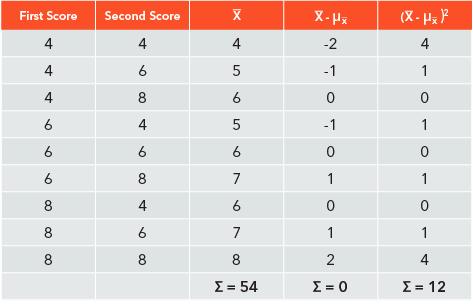Figure 6.20: Sample means, deviations, and squared deviations from all possible samples of n = 2 from the example population

The mean of the distribution is the average, or mean, of the sample means. It is calculated in this example as

Thus, the “mean of the means” is 6, and has all the properties of the mean presented earlier. Note that this is the same as the population mean. The standard error of the mean, SEM, is calculated in this example as

Therefore, the distribution of sample means for n = 2 from this population has a µ = 6 and a σx̄ = 1.15.

6.20

Which of the following symbols is used to represent the mean of the distribution of sample means?

A

$\mu$

B

$\bar{x}$

C

σ$_\bar{x}$

D

μ$_\bar{x}$

6.21

A distribution of sample means gives us $\_\_\_\_\_\_\_\_\_$ possible sample means, and the frequency with which they occur.

6.22

Which of the following are not possible means from the following population [12, 14, 18, 18, 20] when the sample size is 2? (Select all that apply)

A

17

B

10

C

16

D

25

## The Central Limit Theorem

Establishing the distribution of sample means by the sampling method can be extremely time-consuming and not a very productive use of our time. Fortunately, we again can rely on the hard work of mathematicians to help us. As distributions of the sample mean are made of all possible means of samples for a single sample size n that are drawn from a given population, the distribution of sample means and the population from which the samples are selected are related. The relationships are systematic and are presented in what mathematicians call the central limit theorem, the cornerstone to most statistical procedures. To use the central limit theorem, we need only to know the mean μ and standard deviation σ of the population of scores the means come from, and the sample size n. We do not actually have to select the samples and record the means.

### The central limit theorem has three parts

• The distribution of sample means approaches a normal curve as n increases to infinity.
• The mean of the distribution of sample means has the same value as the mean of the known population. μ  = μ
• The standard error of the mean is the standard deviation of the known population divided by the square root of n.

You can experiment with the graph below to see the effect of changing the size of the samples and the number of samples on the shape of individual samples as compared to the distribution of means. You may want to come back to this demonstration again after seeing additional distributions in upcoming chapters.This Content is Locked
Only a limited preview of this text is available. You'll need to sign up to Top Hat, and be a verified professor to have full access to view and teach with the content.

### The Shape of the Distribution of Sample Means

Without doing the underlying mathematical work, the central limit theorem tells us about the three important parts that describe a distribution: the shape, central tendency, and variability. The first point of the central limit theorem, that the distribution will be normal as n approaches infinity, tells us that the properties of the normal distribution that have been discussed earlier also apply to the distribution of sample means. This includes the probabilities that are in the Standard Normal Table.

The part about “as n approaches infinity” can be confusing and intimidating, but it really only matters when the shape of the population of raw scores is unknown or not normally distributed. If the original population of raw scores is known to be normally distributed, then the distribution of the sample means is also normally distributed regardless of the size of the sample.

If the distribution of raw scores is not normally distributed, then we need a reasonable sample size in order to obtain a distribution of sample means that is normally distributed. If the sample size is too small, the distribution of the sample means will not be normally distributed, and the values in the Standard Normal Table will be inaccurate. Therefore, provided that n is large enough, the Standard Normal Table can be used for sample means just as we have been using it for individual data-points when the raw values are not normally distributed. This begs the question “what is large enough?” Notice that the theorem states that “as n increases”…this says that the larger n is, the closer to the normal curve the distribution of sample means is. This implies that the distribution of sample means is never really a true normal curve. Regardless, once the sample size gets to 30 data-points, the differences between the distribution of sample means and a true normal curve are not substantial and the Standard Normal Table can be used with confidence.

Notice that this first point of the central limit theorem does not make reference to the shape of the distribution of raw scores. This is important because it tells us that no matter how the population of individual scores is distributed, with n ≥ 30, the distribution of sample means is normally distributed. The shape of the raw values really only matters when n < 30. In this chapter, we have considered distributions of raw values that are normally distributed, but not all are, so it is important to examine the shape of the raw score distribution when the sample size is less than 30.

### The Parameters of the Distribution of Sample Means

The second and third points of the central limit theorem are also important but need less explanation. The formulas provide a convenient way to determine the mean and standard error of the distribution of the sample means. One important thing to note is that the standard error of the mean will always be less than the standard deviation. Sample means present the center of a sample, so the minimum and maximum of each sample have less influence when the sample is described by the mean. Because of this, means of samples drawn from the same population are more similar than the raw values. The formula will always give a σx̄ that is smaller than σ, telling us that the distribution of sample means is less variable than the distribution of raw scores from which it is drawn.

### Examples

Example 1: Food Preparation

Suppose that Dr. Smith, a sociologist studying family life, uses census data to determine that the average family spends 40 minutes per day preparing food with a standard deviation of 28. She is working with a sample of 100 families. What are the properties of the distribution of sample means for samples of 100 families? According to the central limit theorem, the distribution can be considered to be normally distributed as the sample size is well above 30. The mean of the distribution of sample means is also 40, as it is the same value of the distribution from which it is drawn. The standard error can be found by using the formula:

The standard error of the mean, the variability of the distribution of sample means, for this example is 2.8. Therefore, the distribution of sample means for samples of 100 families is normally

Thus, by the central limit theorem, we know that the average mean of all possible samples of n = 100 is 40 minutes. As the distribution of sample means is normally distributed, 68.2% of sample means are within 2.8 minutes (± 1 standard deviation) of 40.

Example 2: Food Wastage

Suppose that data on households in the U.S. has found that the average household discards 640 lbs. of food each year, with a standard deviation of 30 lbs. What is the distribution of sample means for samples of n = 50 families? Using the central limit theorem, we know that the distribution of sample means will be:

Normally distributed, with μ =  μ = 640, and

Again, without having to actually obtain all the possible values for the sample mean, the central limit theorem provides the characteristics of the distribution of sample means. Here, we know that the average mean of samples of n = 50 is 640 lbs. and the standard error of 4.24 tells us that 68.2% of all sample means are between 635.76 lbs. and 644.24 lbs. (640 +/- 4.24).

6.23

The mean of a population of IQ scores is 100. What is the mean of the distribution of sample means for samples of size 64?

6.24

For a population of IQ scores with µ = 100 and σ = 16, rank the following sample sizes from greatest to least in terms of the resulting σ$_{x̄}$.

A

$n$ = 25

B

$n$ = 15

C

$n$ = 18

D

$n$ = 10

6.25

For a population of IQ scores with µ = 100 and $\sigma$ = 16, match the sample sizes with the value of σ x̄ that will result according to the central limit theorem.

Premise
Response
1

10

A

5.06

2

16

B

2.70

3

22

C

4

4

35

D

1.85

E

3.41

## Finding Probabilities for Sample Means

When we wanted to find the probability associated with a certain value earlier, we used the z-score formula:

This formula is appropriate only for use with raw data. It converts the difference between the raw value to a z-score using the mean and standard deviation from its population. For sample means, we must use a formula that reflects the distribution of sample means. It is:

To convert a sample mean to a z-score, we take the difference between the sample mean (x̄) and the mean of the distribution of sample means (μx̄ ) and divide that by the standard error of the mean (σx̄ ). As you can see, the z-score formula is just updated here for the distribution of sample means. It looks very similar, but the symbols do represent different numbers, with the values provided by applying the central limit theorem to the parameters of the population of raw values.

Example: Food Preparation

Finding the probability of a sample mean starts with the central limit theorem. In the previous example, Dr. Smith found that the mean food preparation time for families was μ = 40 with a standard deviation σ = 28. The central limit theorem indicated that the distribution of sample means for samples of = 100 are normally distributed with μx̄   = 40 and σx̄ = 2.8. Dr. Smith is interested in the probability of obtaining a sample with a mean less than 36 minutes. The z-score for a sample mean of 36 minutes can be found by using the z-score formula for sample means, and using the values derived using the central limit theorem:

The z-score tells us that a sample mean of 36 is 1.43 standard deviations below the mean of the distribution of all possible sample means. We can now use the Standard Normal Table to see what proportion of the samples means fall below this point. The relevant section of the Standard Normal Table is presented here.

• Locate the z-score in tenths in the left column. This is the row labeled -1.4 for our score of -1.43.
• Move across to the right to the column with the correct hundredths value. For our score, this is the 0.03 column.
• The value at the intersection of the row and column is the area to the left of that z-score in the normal distribution curve. The value is .0764.Figure 6.21: Locating the probability associated with z = -1.43 in the Standard Normal Table​​​

The proportion of the population that is below a z-score of -1.43 is .0764. We can say that there is a 7.64% chance that Dr. Smith will observe a mean food preparation time that is less than 36 in her sample of 100 families.

### Area Above a z-Score

Recall that we can subtract the area to the left of the z-score from 1.0000 to obtain the area above that z-score. For this example, with a sample mean score of 36 and corresponding z-score of -1.43, we can subtract the area to the left of the z-score from 1.0000 to get the proportion of the section above the z-score:

Thus, 92.36% of all samples of n = 100 will have means greater than 36 minutes. Dr. Smith has a much greater chance of obtaining a sample mean greater than 36.

### Area Between a z-Score and the Mean

We can also learn about how much of the population is between the z-score and the mean. As we learned earlier, 50% of the distribution lies below or above the mean. Thus, using the same logic, we can subtract the area to the left of our z-score of -1.43 from .5000 (50%) to obtain the area between the z-score and the mean.

Therefore, 42.36% of all possible sample means with n =100 will be between a sample mean of 36 minutes and the sampling distribution mean of 40 minutes.

6.26

Suzanne needs to find the probability that the mean verbal IQ of a sample of 45 students at her school will be 110 or greater. Which formula should she use?

A

$Z$= $\frac{x-\mu}{\sigma}$

B

$Z$= $\frac{\bar{x}-\mu_{\bar{X}}}{\sigma_{\bar {x}}}$

6.27

Which possible sample mean listed below will be larger than most other possible sample means for the data above?

A

65

B

78

C

88

## The Normal Approximation to a Binomial Distribution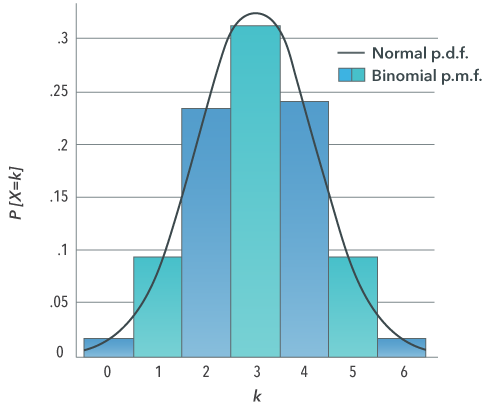Figure 6.22: Binomial probability mass function and normal probability density function approximation for n = 6 and p = 0​​

Another use of the normal distribution is that it can make your life easier. When n is small, i.e., there are not many trials involved, it is easy enough to use the binomial formula to calculate the probability of a given number of successes in n trials. When n is large, however, the procedure becomes less practical because of the number of calculations involved. In this case, it is more convenient to use a normal approximation to the binomial.

The normal approximation to the binomial can be used if the binomial random variable x can be assumed to follow a normal distribution. It is approximately normal if the product of the number of trials n and the probability of a success and of a failure on a given trial are both equal to five or more. The two distributions are not exactly the same but are close enough that the normal approximation can be used in place of the binomial, making the calculations easier. We need to translate the number of independent trials and probability of success or failure in a single trial into values for μ and σ. The formulas for doing so are: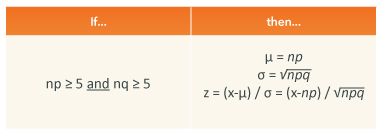Figure 6.23: The Normal approximation of the binomial distribution​​

Note: n represents the number of independent trials, p is the probability of a success in a single trial, and q is the probability of a failure in a single trial (q = 1 - p).

## Applying a Continuity Correction

Before we can calculate the z-score, there is an adjustment to the number of independent observations, x, that needs to be made. A binomial distribution is discrete, consisting of numbers of successes and failures expressed as integers (whole numbers). Discrete variables represent measurements that are indivisible, with no values possible between the measurements. The number of heads on 10 coin tosses fits this definition; there is no such thing as 7.5 heads in 10 tosses of a coin. The normal distribution, on the other hand, is a continuous distribution. Continuous variables work differently. Continuous variables allow an infinite number of observations between two observations. It is as though a discrete variable has height, but no width, while a continuous variable has both height and width.

In order to more closely approximate a normal distribution, a correction needs to be made to the discrete values in the binomial in the form of a continuity correction. Each discrete value in the binomial distribution actually represents the midpoint of the score. If you visualize each score as a histogram bar, the actual boundaries of each score are x - 0.5 and x + 0.5. To apply the correction, simply add 0.5 to the observation for the highest value and/or subtract 0.5 from the observation for the lowest.

### Examples of Applying the Correction

The correction is to either add or subtract 0.5 of a unit from each discrete x-value. This fills in the gaps to make it continuous. This is very similar to expanding of limits to form boundaries, as was done with group frequency distributions.​Figure 6.24: Continuity correction for x = 6​​

As the normal distribution is based on continuous variables, we have to take this difference into consideration by using the real limits of the x-value. The real limit that is appropriate depends upon the section of the distribution in which we are interested. The proper real limit to use depends on the area relative to x that is needed. In the table above, the discrete variable observation of 6 is between 5.5 and 6.5 on a continuous distribution. When the continuity correction values of 6 are placed on the normal distribution, it creates three areas of the curve. One is the area below 5.5, another is the area above 6.5, and the thin section between 5.5 and 6.5 are the things that we will call “5.0”. This thin section must be included when using the Standard Normal Table to determine the areas that include 6 (x ≤ 6 and x ≥ 6).

Example: Probability of Developing Shingles

According to a recent medical report, 35% of the population over 45 years of age will develop shingles, a viral rash related to chickenpox. If you were to randomly select 100 individuals over 45 from the general population, what is the probability that at least 40 will develop shingles?

• n = 100, p = .35, q = .65
• np = 35 and nq = 65. Since both np and nq are ≥ 5, you can use the normal distribution to approximate the distribution of x, the binomial variable.
• μ = np = 35 σ = √npq = √22.75 = 4.7
•  Apply the continuity correction: P(x ≥ 39.5)
• z = (x – μ) / σ = (39.5 – 35) / 4.77 = 4.5 / 4.77 = 0.94
• Use the standard normal table: The tabled entry is 0.8264, so P(x ≤ 39.50) = 0.8264, making P(x ≥ 39.5) = 0.1736
• The probability that at least 40 persons will develop shingles is .1736, or ~.17.

Example: Probability That 40 Develop Shingles

Note that if the question above had asked for the probability that exactly 40 would develop shingles, the method changes somewhat to accommodate that difference.

Step 1: n = 100, p = .35, q = .65

Step 2: np = 35 and nq = 65. Since both np and nq are ≥ 5, you can use the normal distribution to approximate the distribution of x, the binomial variable.

Step 3:

Step 4: Apply the continuity correction: P(x = 40) = P(39.5 ≤ x ≤ 40.5)

Step 5:

Step 6: Use the standard normal table:

Step 7: The probability that 40 persons will develop shingles is .0485, or ~.05.

6.28

In a binomial distribution, p = .15 and n = 50. Can the normal approximation to the binomial distribution be used?

A

Yes

B

No

6.29

In a binomial distribution, p = .70 and n = 25. Can the normal approximation to the binomial distribution be used?

A

Yes

B

No

6.30

Match each discrete variable with the appropriate continuity correction to use with the normal distribution.

Premise
Response
1

x > 25

A

x < 24.5

2

x ≥ 25

B

x ≥ 24.5

3

x < 25

C

x ≤ 25.5

4

x ≤ 25

D

x > 25.5

## Case Study: Blood Pressure and Age

Data is presented by Wright, Hughes, Ostchega, Yoon, & Nwanko (2011) on the blood pressure of American adults collected from 2001-2008. The data was collected on 19,921 adults through the National Health and Nutrition Examination Survey. For this example, we will treat this very large sample as a population. Blood pressure is reported as mmHg (millimeters of Mercury) and is two numbers, systolic and diastolic. Systolic blood pressure is the pressure in arteries when the heart beats, and diastolic is the pressure in arteries between beats. The analysis breaks down hypertension (high blood pressure) by demographic characteristics and treatment status.

Table 2 in the paper presents the results for systolic blood pressure by age group, ethnicity, and hypertensive treatment status. The means and standard errors for each group are presented. While the standard deviation is not directly presented, it can be determined by using the size of each group (N) and the standard error in the formula for the standard error from the central limit theorem, and solving for the standard deviation σ. The calculation will only yield an estimate of σ here as the standard error is given to only one decimal. The data below is drawn from the study. The information is presented below:Figure 6.25: Systolic blood pressure by age group​

### Case Study Question 6.01

Case Study Question 6.01

Assuming that the populations these samples are drawn from are normally distributed, which seems reasonable given the figures in the paper, what are the median and mode for each age-group population?

Click here to see the answer to Case Study Question 6.01.

### Case Study Question 6.02

Case Study Question 6.02

Hypertension is diagnosed when a person’s systolic blood pressure is greater than 140 mm Hg. To find the proportion of individuals in each population who have hypertension, we need to convert 140 to a z-score. Which formula from the chapter would be appropriate? Would the standard deviation or standard error be used?

Click here to see the answer to Case Study Question 6.02.

### Case Study Question 6.03

Case Study Question 6.03

Without doing any calculation, how will the proportion that would be diagnosed as hypertensive be different for each age group population? Which age group will have the largest proportion that is hypertensive? Which age group will have the smallest proportion that is hypertensive?

Click here to see the answer to Case Study Question 6.03.

### Case Study Question 6.04

Case Study Question 6.04

Find the proportion of each population that has hypertension: a. What proportion of the population aged 18-39 has hypertension? b. What proportion of the population aged 40-59 has hypertension? c. What proportion of the population aged 60 and over has hypertension?

Click here to see the answer to Case Study Question 6.04.

### Case Study Question 6.05

Case Study Question 6.05

What does the larger variability for the oldest age group suggest about the health of the population?

Click here to see the answer to Case Study Question 6.05.

## Pre-Class Discussion Questions

### Class Discussion 6.01

Class Discussion 6.01

What is the benefit of using the normal distribution to approximate the binomial distribution? What is a disadvantage?

Click here to see the answer to Class Discussion 6.01.

### Class Discussion 6.02

Class Discussion 6.02

A video in the chapter presented how normal curves can be seen in wear patterns in the world around us. What are some other examples of such wear patterns that could be found on a college campus?

Click here to see the answer to Class Discussion 6.02.

### Class Discussion 6.03

Class Discussion 6.03

What happens when one uses the central limit theorem to find the distribution of sample means, but n is less than 30?

Click here to see the answer to Class Discussion 6.03.

### Class Discussion 6.04

Class Discussion 6.04

For the PSY 100 class distribution with µ = 70 and σ = 10, what is the exam raw score that corresponds to a z-score of 0.79? What is the exam raw score that corresponds to a z-score of -0.79? Why are these exam raw scores different? How are these scores the same?

Click here to see the answer to Class Discussion 6.04.This Content is Locked
Only a limited preview of this text is available. You'll need to sign up to Top Hat, and be a verified professor to have full access to view and teach with the content.

## Answers to Case Study Questions

### Answer for Case Study Question 6.01

If the populations from which these very large samples have been drawn are normally distributed, then the medians and modes for each will be very close to the mean for each. In a perfect normal distribution, all three measures of central tendency are the same value. Real distributions tend not to be perfect, so the medians and modes will be very similar in value to the means.

Click here to return to Case Study Question 6.01.

### Answer for Case Study Question 6.02

The z-score formula that is appropriate is the formula for individual scores, which uses the population standard deviation. It is:

Click here to return to Case Study Question 6.02.

### Answer for Case Study Question 6.03

The percentage of people in each age group diagnosed with hypertension will be larger for the older age groups. The definition of hypertension is fixed at a systolic blood pressure greater than 140 mm Hg. As the mean systolic blood pressure increases with age, it comes closer to 140 mm Hg. The closer 140 mm Hg is to the mean, a larger part of the distribution will be above 140 mm Hg and be diagnosed as hypertensive.

Click here to return to Case Study Question 6.03.

### Answer for Case Study Question 6.04

a) The 18-39 age group has a mean blood pressure of 115 mm Hg with a standard deviation of 17.52 mm Hg. The z-score for 140 mm Hg is +1.43. The Standard Normal Table tells us that .9236 of the population falls below this z-score. Therefore, the proportion above the z-score can be found by subtracting this proportion from 1.0000.

Therefore, the proportion of the 18-39 age group that is hypertensive is .0746

b) The 40-59 age group has a mean blood pressure of 123 mm Hg with a standard deviation of 22.90 mm Hg. The z-score for 140 mm Hg is +0.74. The Standard Normal Table tells us that .7704 of the population falls below this z-score. Therefore, the proportion above the z-score can be found by subtracting this proportion from 1.0000.

The proportion of the 40-59 age group that is hypertensive is .2296.

c) The over 60 age group has a mean blood pressure of 136 mm Hg with a standard deviation of 40.06 mm Hg. The z-score for 140 mm Hg is +0.10. The Standard Normal Table tells us that .5398 of the population falls below this z-score. Therefore, the proportion above the z-score can be found by subtracting this proportion from 1.0000.

The proportion of the 40-59 age group that is hypertensive is .4602.

Click here to return to Case Study Question 6.04.

### Answer for Case Study Question 6.05

The larger systolic blood pressure variability suggests that the overall health of people in this age group varies much more than in the younger age groups.

Click here to return to Case Study Question 6.05.

## Answers to Pre-Class Discussion Questions

### Answer to Class Discussion 6.01

A benefit is that it saves calculation when the probability of many individual binomial outcomes needs to be computed, such as when n is large. A disadvantage is that as an approximation, there will be some difference from the probabilities obtained with the normal curve from the exact binomial probabilities. Another disadvantage is that one must remember to use the continuity correction.

Click here to return to Class Discussion 6.01

### Answer to Class Discussion 6.02

Wear patterns can be seen on old stairs, and paths students take across grassy areas. These are inverted normal curves. Cafeteria trays may be worn more in the middle. Treadmills in the fitness center also are worn more in the middle, and actually may form two inverted normal curves with one for the right foot and one for the left foot. Hallways floors may get wetter in the center than along the walls on rainy days.

Click here to return to Class Discussion 6.02

### Answer to Class Discussion 6.03

It depends upon the nature of the distribution of raw scores. If the distribution of raw scores is normally distributed, then there is no problem and the distribution of sample means will be normal, and the Standard Normal Table can be used. If the distribution of raw scores is not normally distributed, then the distribution of sample means will differ from a normal curve. In this case, the probability entries in the Standard Normal Table will not be accurate as the table assumes a normal curve

Click here to return to Class Discussion 6.03

### Answer to Class Discussion 6.04

For the z-score of 0.79, x = 77.9. For the z-score of -0.79, x = 62.1. The exam raw scores are different because one is above the mean (positive z-score) and one is below (negative z-score). The exam raw scores are similar in that they are the same difference from the mean.

Click here to return to Class Discussion 6.04

### Image Credits

 Image courtesy of Resurrection Pleasant Hill CA under CC BY-SA 3.0.